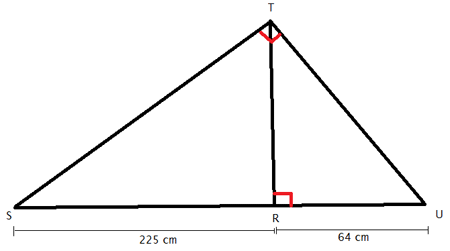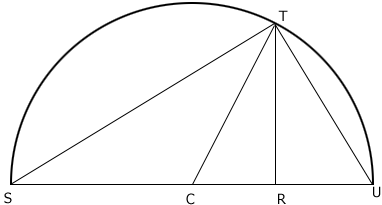SEARCH HOMEMath Central Quandaries & QueriesI am having a lot of difficulties with this question. Name: Jora Subject: Math Who are you: Student Thank you.Hi Jora,

You sent us this diagram but no question.Use the fact that the right angle STU is inscribed in a semicircle whose diameter is SU. (The radius CT from the center C of the circle to the point T is, of course, half the diameter.)

Chris

Jora wrote back

Sorry for not asking the question, The question is what is the length from TR?

Jora

Were you able to use Chris' suggestion to find the length of TR?

Harley

I have tried but I couldn't really understand what he meant.

Jora

If you know the fact that Chris mentioned then C, the midpoint of SU is the center of a circle that passes through U, T and S.Since CS, CT and CU are all radii of the circle they all have the same length,

Consider triangle TCR. It is a right triangle and you know the lengths of two sides so Pythagoras Theorem will give you the length of the third side.

HarleyMath Central is supported by the University of Regina and The Pacific Institute for the Mathematical Sciences.